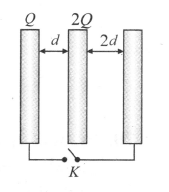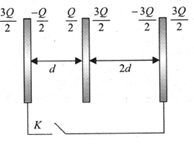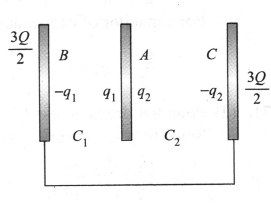Properties of conductor
Question

# Three large plates are arranged as shown. How much charge will flow through the key k if it is closed?Moderate
Solution

## Before closing the switchAfter closing the switch${q}_{1}+{q}_{2}=2Q$${V}_{AB}={V}_{AC}\text{\hspace{0.17em}}or\text{\hspace{0.17em}\hspace{0.17em}}\frac{{q}_{1}}{{C}_{1}}=\frac{{q}_{2}}{{C}_{2}}$$\text{\hspace{0.17em}\hspace{0.17em}}\frac{{q}_{1}}{{q}_{2}}=\frac{{C}_{1}}{{C}_{2}}=2\text{\hspace{0.17em}\hspace{0.17em}}or\text{\hspace{0.17em}\hspace{0.17em}}{q}_{1}=2{q}_{2}$Solving, we get  ${q}_{1}=\frac{4Q}{3},\text{\hspace{0.17em}\hspace{0.17em}}{q}_{2}=\frac{2Q}{3}$Charge flown through K is$-\frac{Q}{2}-\left(-{q}_{1}\right)=-\frac{Q}{2}+\frac{4Q}{3}=5Q/6$

Get Instant Solutions